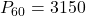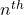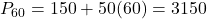## Consider a population that grows to the recursive rule Pn= Pn-1 + 50, with initial population Po = 200 (a) Use the recursive for

Question

Consider a population that grows to the recursive rule Pn= Pn-1 + 50,
with initial population Po = 200
(a) Use the recursive formula to find: p1 and p2
(b) Find an explicit formula for the population.
(c) Use the explicit formula to find P60

in progress 0
2 weeks 2021-08-29T00:08:11+00:00 1 Answers 0 views 0

a)   P₁  = 250 and    P₂ = 300

b) The explicit formula   Pₙ = 150+ 50 n

c)Step-by-step explanation:

Step(i):-

Given that Pₙ = pₙ₋₁ +50 and P₀ = 200

Put n =1

P₁ = P₀+50 = 200+50 = 250

Put n =2

P₂ = P₁+50 = 250+50 = 300

Put n=3

P₃ = P₂+50 = 300+50 = 350

and so on

The sequence     200,250,300,350,..

Step(ii):-

The sequence     200,250,300,350,.. is an arithmetic sequence

The explicit formula

Pₙ = a+(n-1)d

Pₙ = 200+(n-1)50

Pₙ = 200+ 50 n -50

Pₙ = 150+ 50 n

The explicit formula   Pₙ = 150+ 50 n

c)

Theterm of the sequence

Pₙ = 150+ 50 n

put n=60